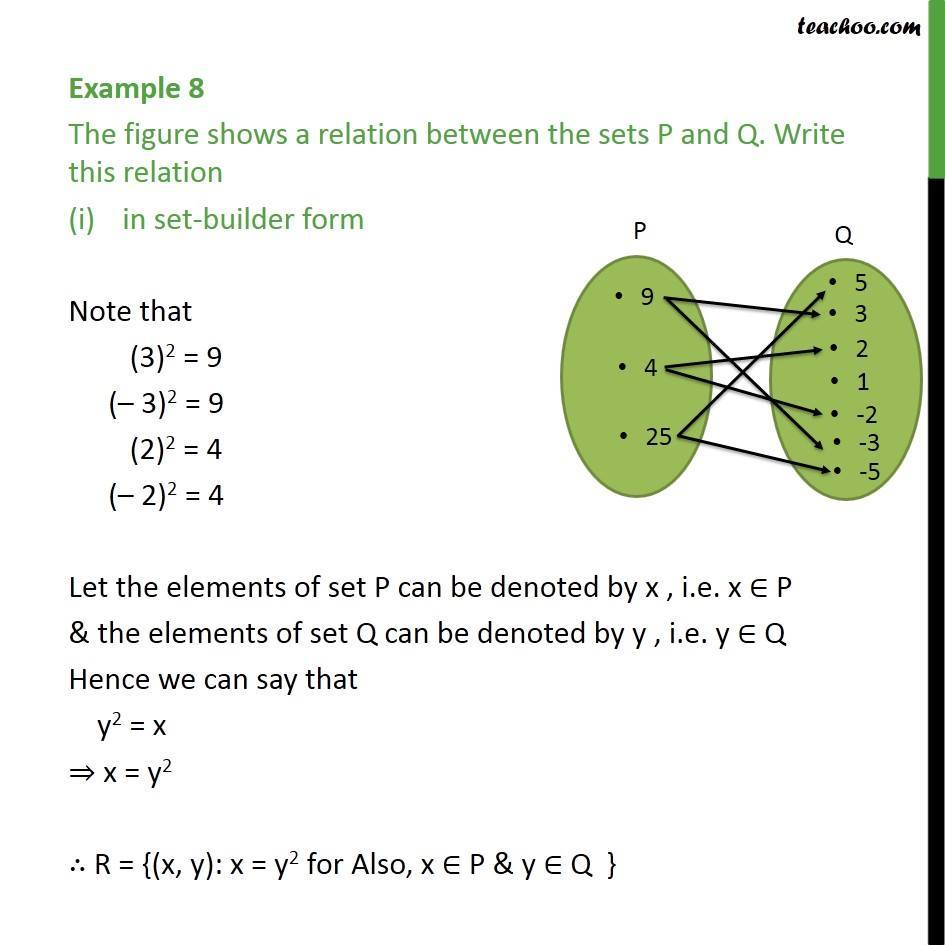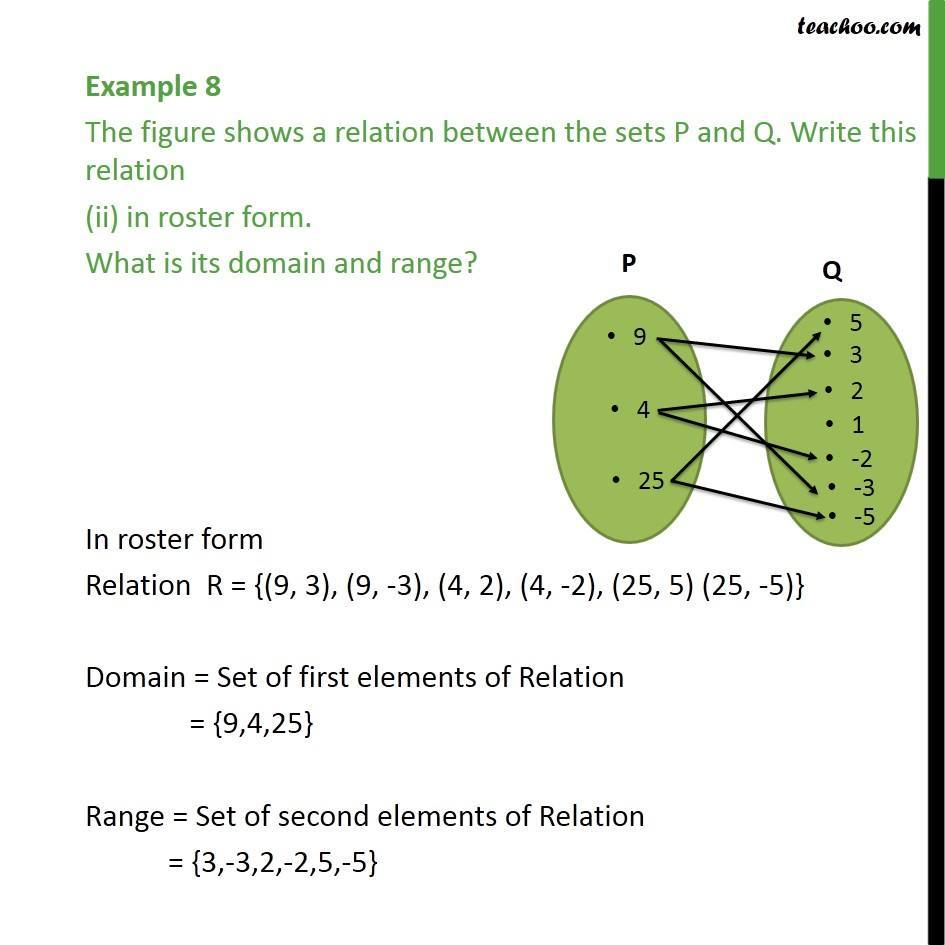1. Chapter 2 Class 11 Relations and Functions
2. Concept wise
3. Finding Relation - Arrow Depiction given

Transcript

Example 8 The figure shows a relation between the sets P and Q. Write this relation in set-builder form Note that (3)2 = 9 (– 3)2 = 9 (2)2 = 4 (– 2)2 = 4 Let the elements of set P can be denoted by x , i.e. x ∈ P & the elements of set Q can be denoted by y , i.e. y ∈ Q Hence we can say that y2 = x ⇒ x = y2 ∴ R = {(x, y): x = y2 for Also, x ∈ P & y ∈ Q } Example 8 The figure shows a relation between the sets P and Q. Write this relation (ii) in roster form. What is its domain and range? In roster form Relation R = {(9, 3), (9, -3), (4, 2), (4, -2), (25, 5) (25, -5)} Domain = Set of first elements of Relation = {9,4,25} Range = Set of second elements of Relation = {3,-3,2,-2,5,-5}

Finding Relation - Arrow Depiction given